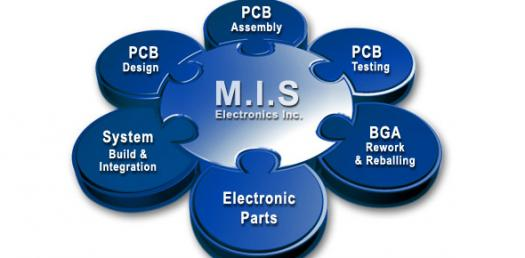# MIS 502 Ch 4 Quiz Drilled Down -

31 Questions | Total Attempts: 63SettingsMIS 502 CH 4 Quiz Drilled Down -

• 1.
A(n) load instruction copies data from one memory location to another.
• A.

True

• B.

False

• 2.
The CPU always determines the next instruction to fetch by looking in the instruction pointer.
• A.

True

• B.

False

• 3.
A reduced instruction set computing (RISC) processor does not directly implement instructions that combine data movement and manipulation.
• A.

True

• B.

False

• 4.
In many CPUs, a register called the ____ stores condition codes, including those representing processing errors and the results of comparison operations.
• A.

Program status word

• B.

Instruction register

• 5.
Branch prediction improves CPU performance by dividing instruction execution into stages and overlapping execution of multiple instructions in different stages.
• A.

True

• B.

False

• 6.
With respect to two&#039;s complement values, which of the following is NOT a complex instruction than can be implemented by a sequence of more primitive instructions?
• A.

Divide

• B.

• 7.
A(n) ____ is a template that specifies the number of operands and the position and length of the op code and operands.
• A.

Reduced Instruction Set

• B.

PSW

• C.

Heat sink

• D.

Instruction format

• 8.
In many CPUs, a register called the program status word (PSW) stores condition codes, including those representing processing errors and the results of comparison operations.
• A.

True

• B.

False

• 9.
A CPU with multiple execution units can more efficiently evaluate the formula ((((a + b) x c) - d) / e).
• A.

True

• B.

False

• 10.
If A and B are two&#039;s complement values, the result of subtracting B from A can be computed by XORing A with a string of 1-bits, ADDing one to the result, then ADDing B.
• A.

True

• B.

False

• 11.
The components of an instruction are its op code and one or more ____.
• A.

Boolean values

• B.

Operands

• 12.
A CPU typically implements multiple instruction formats to account for differences among instructions in the number and type of op codes.
• A.

True

• B.

False

• 13.
The address of the next instruction to be fetched by the CPU is held in the instruction register.
• A.

True

• B.

False

• 14.
Which of the following causes the processor to depart from sequential instruction order?
• A.

BRANCH

• B.

MOVE

• C.

SHIFT

• D.

• 15.
Shifting a binary value one bit to the right multiplies the value by 2.
• A.

True

• B.

False

• 16.
The ____ tests the bit values in the source location and places copies of those values in the destination location.
• A.

• B.

MOVE

• C.

STORE

• D.

• 17.
Doubling word size ____ CPU transistor count.
• A.

Less than doubles

• B.

Doubles

• C.

More than doubles

• D.

Has no relationship to

• 18.
A(n) MOVE instruction copies data bits to storage locations.
• A.

True

• B.

False

• 19.
Current fabrication technology is capable of squeezing ____ transistors onto a wafer of silicon approximately one square centimeter in size.
• A.

Under 100 million

• B.

Approximately 100 million

• C.

Approximately 200 million

• D.

Over 300 million

• 20.
A CPU typically implements multiple instruction formats to account for differences among instructions in the number and type of op codes.
• A.

True

• B.

False

• 21.
The components of an instruction are its operand and one or more op codes.
• A.

True

• B.

False

• 22.
A CPU with multiple execution units can more efficiently evaluate the formula (((a + b) x (c + d)) - ((e + f) / (g + h))).
• A.

True

• B.

False

• 23.
The word size of a processor should be ____ the width of the data bus for maximal performance.
• A.

Less than

• B.

No more than

• C.

At least as large as

• D.

Greater than

• 24.
The term pipelining describes instruction execution beyond a conditional branch instruction before the branch condition value is know with certainty.
• A.

True

• B.

False

• 25.
What law predicts that the cost of a manufacturing plant and equipment for the latest generation of microprocessors doubles every four years?
• A.

Moore’s Law

• B.

Rock’s Law

• C.

Shannon’s Law

• D.

Gallium’s Law

Related TopicsBack to top